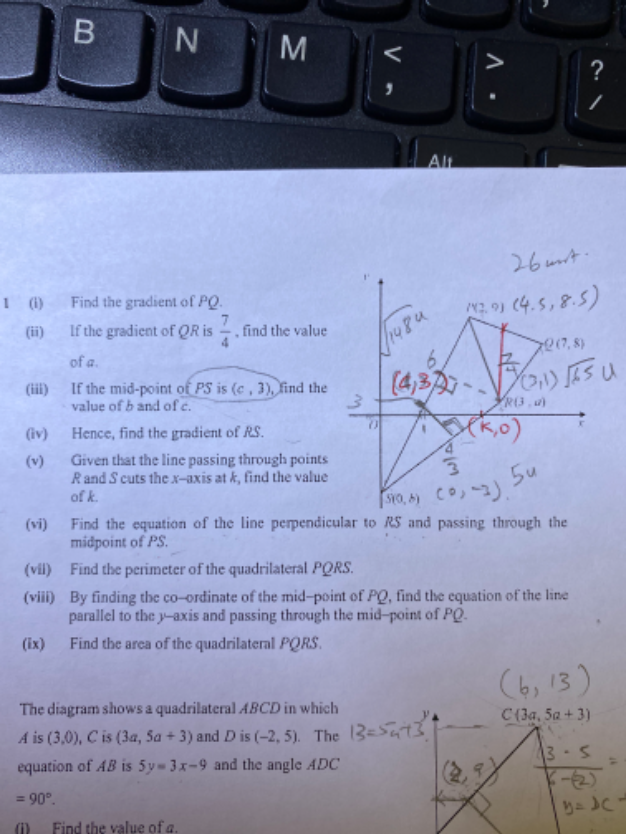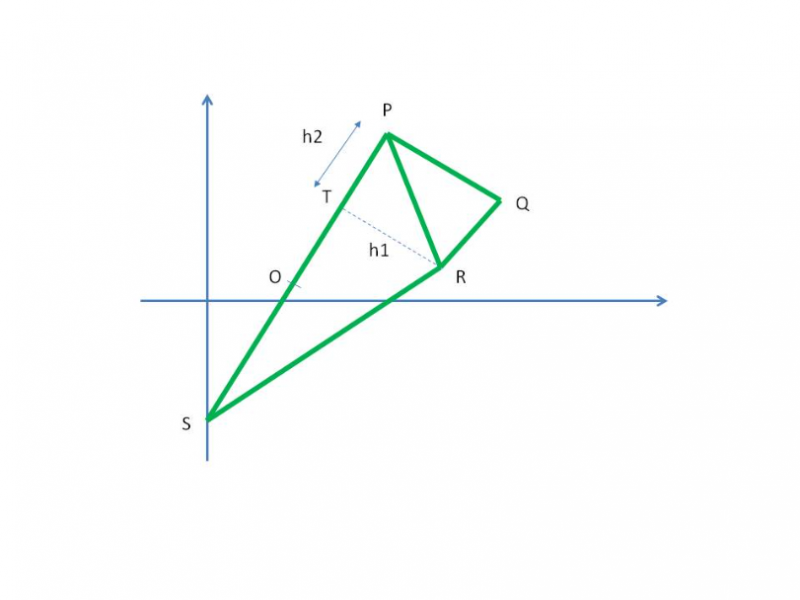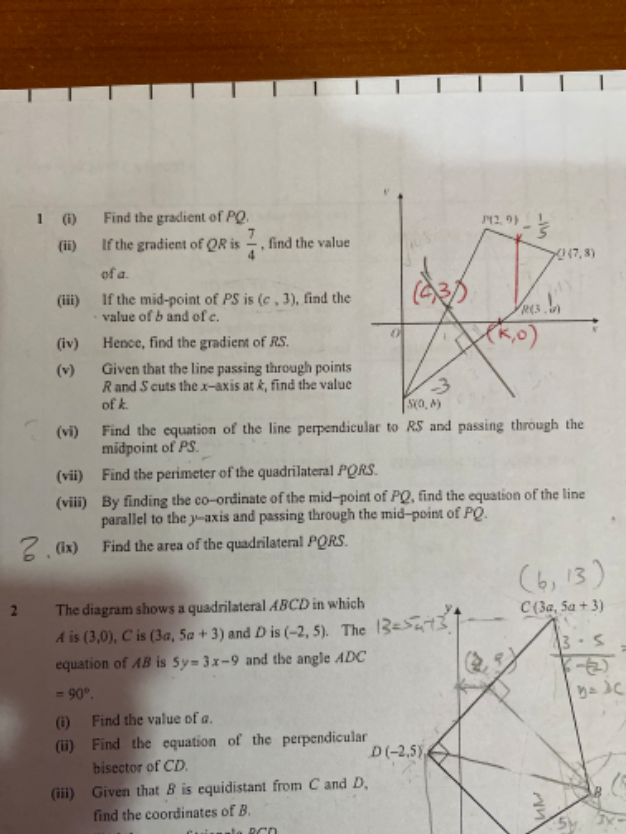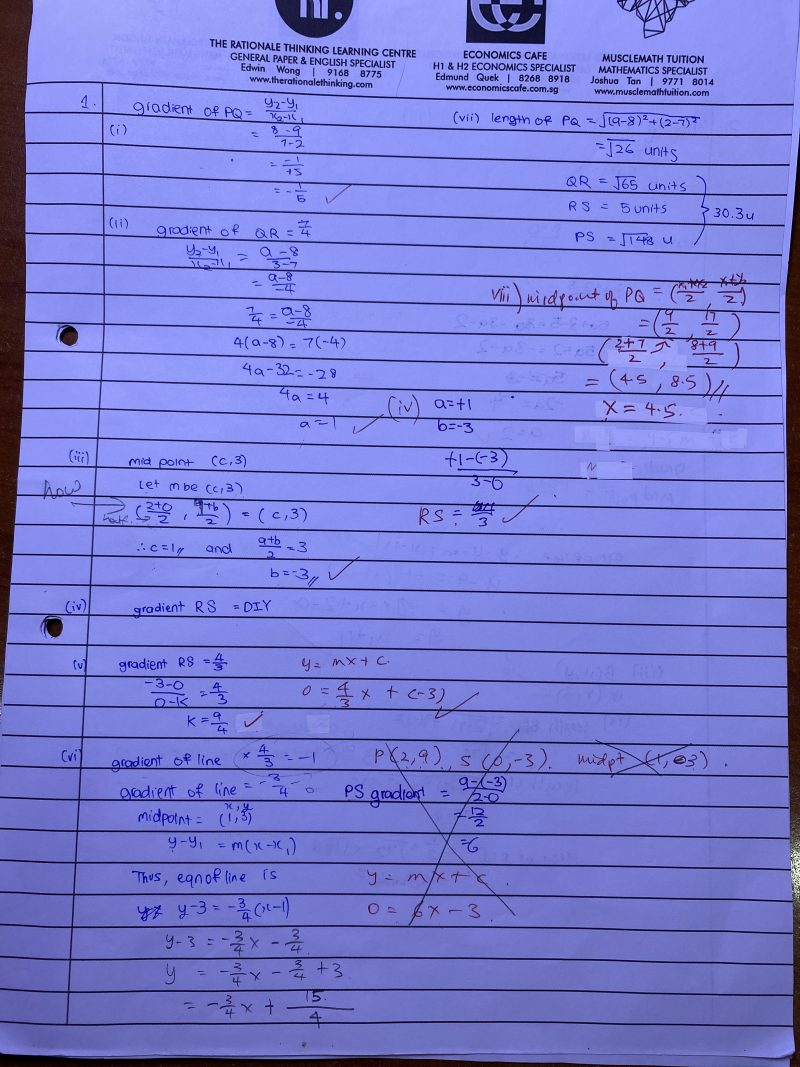# Questionanswer given is 33 1/2 units ^2Hi,

Typically for such questions, the parts are all linked to find the answers.

As your image is quite blur upon magnifying and a lot of scribblings on it, it is quite difficult to figure out what’s going on.

Instead of solving it, let me guide you to solve it since you have all the numbers with you.

Based on my diagram,

1. Can you prove that PS is perpendicular to PQ?  If yes, then h2 is the height for triangle PQR.
2. Based on the co-ordinates  of T and R , you can find h1.
3.  Since you have already found the perimeter of this figure, I would assume you know all the lengths of the sides of this figure.
4. So find area of triangle PSR with height h1 and base PS.
5. Find area of triangle PQR with height h2 and base PQ
6. Add both up will give area of figure.
1 Reply 1 Like1 Reply 1 Like

Sorry about the blur image all the time, i think my iphone 11 resolution not so good. i was analyzing your explanation, I think i have difficulty find the T coordinate to get the h1 and also which one will be the height of triangle TSR although i have the midpoint coordinate.

1 Reply 0 Likes

Since the 4 coordinates are known as found in all the above steps, there is an easy way to do this.

P = (2.9)

Q = ( 7,8 )

R = (3, 1)

S  = (0,-3)

Area of quadrilateral = Area of triangle PQR + Area of triangle PSR   (sq units)

Area of PQR = (1/2) y1(x2x3)+y2(x3x1)+y3(x1x2) ∣   (mod means take only the abs value = +ve)

=(1/2) ∣ 2(8-1) + 7(1-9) + 3(9-8) ∣

= (1/2) | 14 – 56 + 3 |

=(1/2) ( 39) = 19.5

Area of PSR = (1/2) y1(x2x3)+y2(x3x1)+y3(x1x2)

= (1/2) 2(-3-1) + 0(1-9) + 3(9+2)

=(1/2) ∣ -8 + 0 + 36

= (1/2) (28)

=14

Area of quadrilateral = 19.5 + 14 = 33.5 sq units

The area of quadrilateral formula is covered in “coordinate geometry”.

0 Replies 1 Like